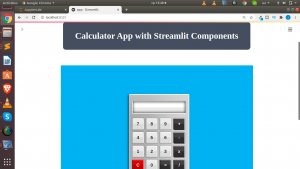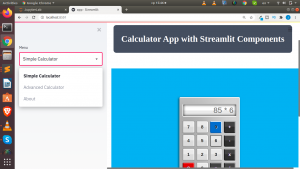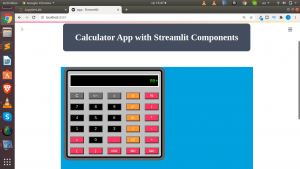# Building A Calculator with Streamlit Components and HTML

Streamlit components have so much potential. With the introduction of the components system in streamlit, we can now build additional features and integrate into the web apps we have been building. You can check out the docs on the components here.

In this tutorial we will explore one of the components  – .html().

We will be using this new feature to build a simple calculator and a more advanced calculator using just HTML,JavaScript and CSS.Let us see the steps we will be using;

• Designing the calculator with HTML,CSS and JS
• Save as html file(Of course)
• Reading the html file using codecs
• Rendering our calculator using .html()

What we will be doing is that – we will first design our calculator using HTML,CSS and JS and then with the help of the component.html() function we will render our calculator in streamlit. There are a lot of tutorials available on how to design either a simple or advanced calculator so that part is sorted out. You can check out Gregory advanced calculator- which is what we will use for the advanced calculator.

The basic logic behind this app is that we are just reading our html files and relying on the .html() to help us render it. Hence streamlit will serve as a server serving our simple calculator.

But to be apply to read our html file we will need to use the python module codecs and then parse the result to streamlit component. We will create a simple function to read,parse and render our html file.

``````def st_calculator(calc_html,width=700,height=500):
calc_file = codecs.open(calc_html,'r')
components.html(page,width=width,height=height,scrolling=False)
``````

You can use this same function for different apps that works with html,css and javascript.

The basic structure of app will consist of  a section for a simple calculator and another section for the advanced calculator. We will create a .py file and name it as app.py and place in the code below.

``````# Core Pkgs
import streamlit as st

# Utils Pkgs
import codecs

# Components Pkgs
import streamlit.components.v1 as components

# Custom Components Fxn
def st_calculator(calc_html,width=700,height=500):
calc_file = codecs.open(calc_html,'r')
components.html(page,width=width,height=height,scrolling=False)

def main():
"""A Calculator App with Streamlit Components"""

components.html(html_temp)

else:
components.html(html_temp)
st_calculator('calculator.html')

if __name__ == '__main__':
main()
``````

We can now run our app as always with

`streamlit run app.py`

Voila – our calculator app is done and it is working.You can see how easy yet powerful the components feature of streamlit in building something very useful.You can also check out the video tutorial below.